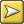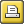﻿ formulae
 FormulaeFor computing collocation strength, we can use

 • the joint frequency of two words: how often they co-occur, which assumes we have an idea of how far away counts as "neighbours". (If you live in London, does a person in Liverpool count as a neighbour? From the perspective of Tokyo, maybe they do. If not, is a person in Oxford? Heathrow?)
 • the frequency word 1 altogether in the corpus
 • the frequency of word 2 altogether in the corpus
 • the span or horizons we consider for being neighbours
 • the total number of running words in our corpus: total tokens

Mutual Information

Log to base 2 of (A divided by (B times C))

where

A = joint frequency divided by total tokens

B = frequency of word 1  divided by total tokens

C = frequency of word 2  divided by total tokens

MI3

Log to base 2 of ((J cubed) times E divided by B)

where

J = joint frequency

F1 = frequency of word 1

F2 = frequency of word 2

E = J + (total tokens-F1) + (total tokens-F2) + (total tokens-F1-F2)

B = (J + (total tokens-F1)) times (J + (total tokens-F2))

T Score

((X divided by total tokens) - X) divided by (square root of (J))

where

J = joint frequency

F1 = frequency of word 1

F2 = frequency of word 2

X = F1 times F2

Z Score

(J - E) divided by the square root of (E times (1-P))

where

J = joint frequency

S = collocational span

F1 = frequency of word 1

F2 = frequency of word 2

P = F2 divided by (total tokens - F1)

E = P times F1 times S

Dice Coefficient

(J times 2) divided by (F1 + F2)

where

J = joint frequency

F1 = frequency of word 1 or corpus 1 word count

F2 = frequency of word 2 or corpus 2 word count

Ranges between 0 and 1.

Log Likelihood

based on Oakes p. 170-2.

2 times (

a Ln a + b Ln b + c Ln c + d Ln d

- (a+b) Ln (a+b)

- (a+c) Ln (a+c)

- (b+d) Ln (b+d)

- (c+d) Ln (c+d)

+ (a+b+c+d) Ln (a+b+c+d)

)

where

a = joint frequency

b = frequency of word 1

c = frequency of word 2

d := frequency of pairs involving neither w1 nor w2

and "Ln" means Natural Logarithm